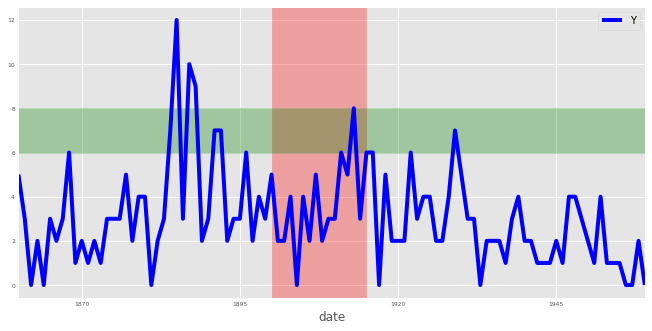import pandas as pd
import numpy as np
import matplotlib.pyplot as plt
import seaborn as sns

plt.rcParams['figure.figsize'] = (10, 5)


## Welcome to the course!

• Time series in the field of Data Science
• Time series are a fundamental way to store and analyze many types of data
• Financial, weather and device data are all best handled as time series

In this exercise, you will read in a time series dataset that contains the number of "great" inventions and scientific discoveries from 1860 to 1959, and display its first five rows.

discoveries = pd.read_csv('./dataset/ch1_discoveries.csv')

# Display the first five lines of the DataFrame

         date  Y
0  01-01-1860  5
1  01-01-1861  3
2  01-01-1862  0
3  01-01-1863  2
4  01-01-1864  0


### Test whether your data is of the correct type

When working with time series data in pandas, any date information should be formatted as a datetime64 type. Therefore, it is important to check that the columns containing the date information are of the correct type. You can check the type of each column in a DataFrame by using the .dtypes attribute. Fortunately, if your date columns come as strings, epochs, etc... you can use the to_datetime() function to convert them to the appropriate datetime64 type:

df['date_column'] = pd.to_datetime(df['date_column'])


In this exercise, you will learn how to check the data type of the columns in your time series data and convert a date column to the appropriate datetime type.

discoveries['date'] = discoveries['date'].astype('object')

print(discoveries.dtypes)

# Convert the date column to a datestamp type
discoveries['date'] = pd.to_datetime(discoveries['date'])

# Print the data type of each column in discoveries, again
print(discoveries.dtypes)

date    object
Y        int64
dtype: object
date    datetime64[ns]
Y                int64
dtype: object


## Plot your first time series

Let's take everything you have learned so far and plot your first time series plot. You will set the groundwork by producing a time series plot of your data and labeling the axes of your plot, as this makes the plot more readable and interpretable for the intended audience.

matplotlib is the most widely used plotting library in Python, and would be the most appropriate tool for this job. Fortunately for us, the pandas library has implemented a .plot() method on Series and DataFrame objects that is a wrapper around matplotlib.pyplot.plot(), which makes it easier to produce plots.

discoveries = discoveries.set_index('date')

# Plot the time series in your DataFrame
ax = discoveries.plot(color='blue');

# Specify the x-axis label in your plot
ax.set_xlabel('Date');

# Specify the y-axis label in your plot
ax.set_ylabel('Number of great discoveries');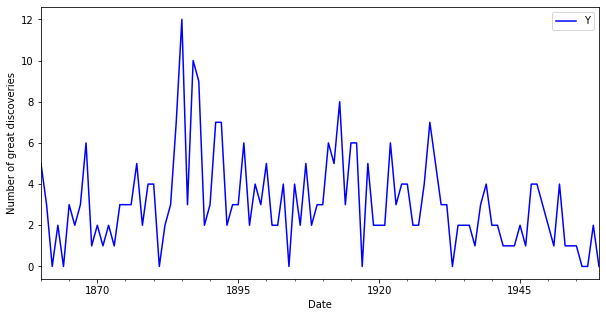### Specify plot styles

The matplotlib library also comes with a number of built-in stylesheets that allow you to customize the appearance of your plots. To use a particular style sheet for your plots, you can use the command plt.style.use(your_stylesheet) where your_stylesheet is the name of the style sheet.

In order to see the list of available style sheets that can be used, you can use the command print(plt.style.available). For the rest of this course, we will use the awesome fivethirtyeight style sheet.

plt.style.use('fivethirtyeight')

# Plot the time series
ax1 = discoveries.plot();
ax1.set_title('FiveThirtyEight Style');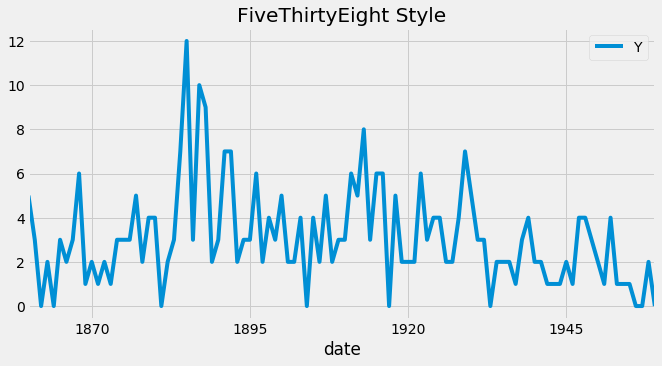plt.style.use('ggplot')
ax2 = discoveries.plot();

# Set the title
ax2.set_title('ggplot Style');### Display and label plots

As you saw earlier, if the index of a pandas DataFrame consists of dates, then pandas will automatically format the x-axis in a human-readable way. In addition the .plot() method allows you to specify various other parameters to tailor your time series plot (color of the lines, width of the lines and figure size).

You may have noticed the use of the notation ax = df.plot(...) and wondered about the purpose of the ax object. This is because the plot function returns a matplotlib AxesSubplot object, and it is common practice to assign this returned object to a variable called ax. Doing so also allows you to include additional notations and specifications to your plot such as axis labels.

ax = discoveries.plot(color='blue', figsize=(8, 3), linewidth=2, fontsize=6);

# Specifiy the title in your plot
ax.set_title('Number of great inventions and scientific discoveries from 1860 to 1959',
fontsize=8);## Customize your time series plot

### Subset time series data

When plotting time series data, you may occasionally want to visualize only a subset of the data. The pandas library provides powerful indexing and subsetting methods that allow you to extract specific portions of a DataFrame. For example, you can subset all the data between 1950 and 1960 in the discoveries DataFrame by specifying the following date range:

subset_data = discoveries['1950-01-01':'1960-01-01']


Note: Subsetting your data this way is only possible if the index of your DataFrame contains dates of the datetime type. Failing that, the pandas library will return an error message.

discoveries_subset_1 = discoveries['1945':'1950']

# Plot the time series in your DataFrame as a blue area chart
ax = discoveries_subset_1.plot(color='blue', fontsize=15);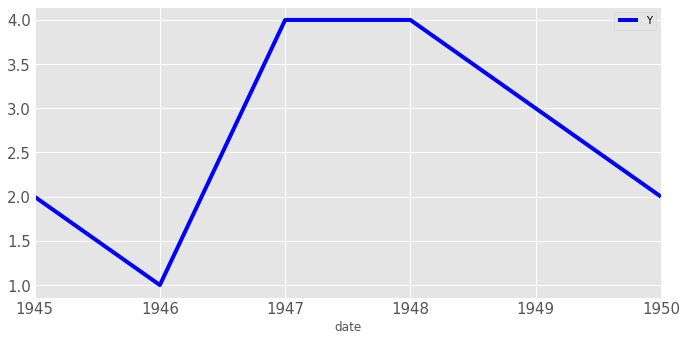discoveries_subset_2 = discoveries['1939':'1958']

# Plot the time series in your DataFrame as a blue area chart
ax = discoveries_subset_2.plot(color='blue', fontsize=15);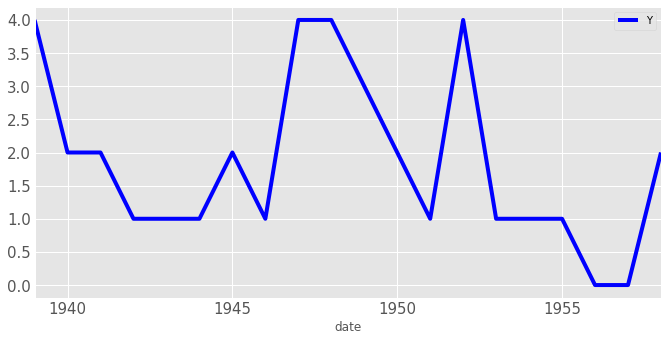### Add vertical and horizontal markers

Additional annotations can help further emphasize specific observations or events. Here, you will learn how to highlight significant events by adding markers at specific timestamps of your time series plot. The matplotlib library makes it possible to draw vertical and horizontal lines to identify particular dates.

Recall that the index of the discoveries DataFrame are of the datetime type, so the x-axis values of a plot will also contain dates, and it is possible to directly input a date when annotating your plots with vertical lines. For example, a vertical line at January 1, 1945 can be added to your plot by using the command:

ax.axvline('1945-01-01', linestyle='--')

ax = discoveries.plot(color='blue', fontsize=6);

# Add a red vertical line
ax.axvline('1939-01-01', color='red', linestyle='--');

# Add a green horizontal line
ax.axhline(4, color='green', linestyle='--');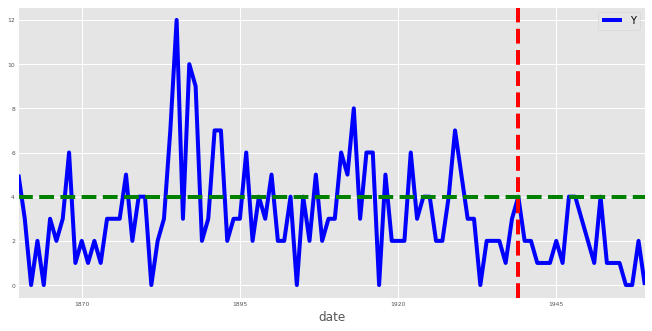When plotting time series data in Python, it is also possible to highlight complete regions of your time series plot. In order to add a shaded region between January 1, 1936 and January 1, 1950, you can use the command:

ax.axvspan('1936-01-01', '1950-01-01', color='red' , alpha=0.5)


Here we specified the overall transparency of the region by using the alpha argument (where 0 is completely transparent and 1 is full color).

ax = discoveries.plot(color='blue', fontsize=6);